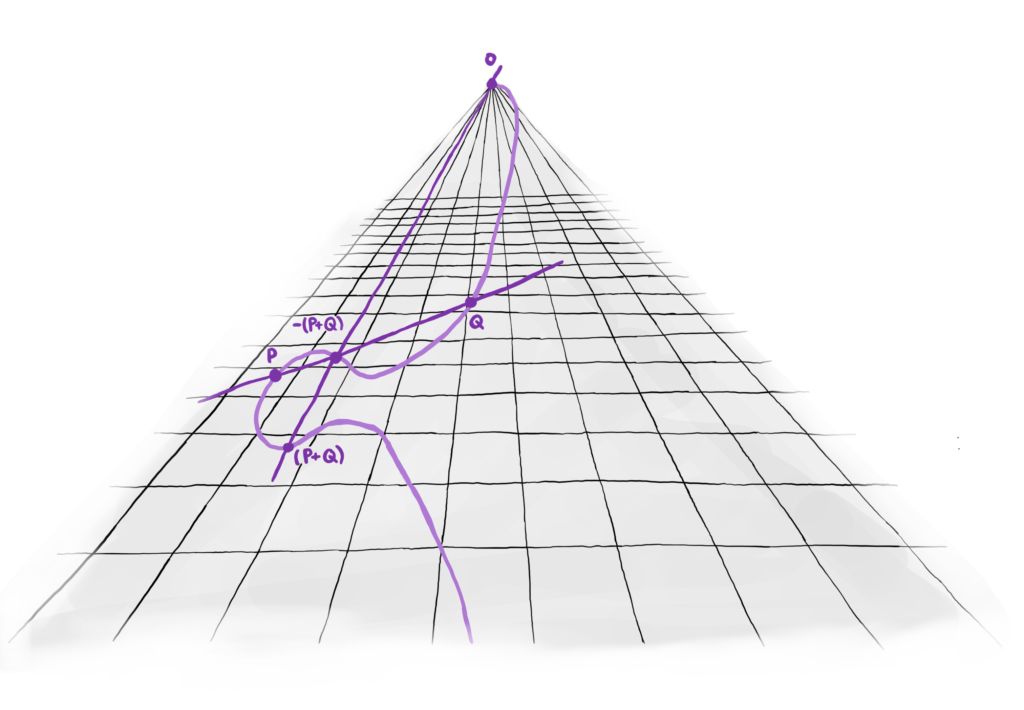# Arithmetic Geometry, Number Theory and Computation

Computation and number theory have gone hand in hand since Mesopotamian times, and the Simons Collaboration on Arithmetic Geometry, Number Theory and Computation, launched in 2018, is taking the relationship into the 21st century with new tools and approaches.A visualization of the elliptic curve group law, an important concept in number theory, on a projected plane. Courtesy of Sachi Hashimoto

Computation and number theory naturally go hand in hand — one of the earliest examples is a Mesopotamian tablet from 1800 BC that lists 15 sets of integers that satisfy the equation a2 + b2 = c2, now known as Pythagorean triples.

The Simons Collaboration on Arithmetic Geometry, Number Theory and Computation continues the legacy of combining computation with theoretical research by focusing on several central problems in the study of numbers and solutions to polynomial equations.

The collaboration, launched in 2018, germinated from a 2015 meeting at the Institute for Computational and Experimental Research in Mathematics at Brown University, titled “Computational Aspects of the Langlands Program” and organized by collaboration investigator John Voight of Dartmouth College and others.

“Our collaboration grew out of the questions: What does computational number theory look like in the 21st century, and what tools should be developed for use by the arithmetic geometry community?” Voight says.

Recent Articles